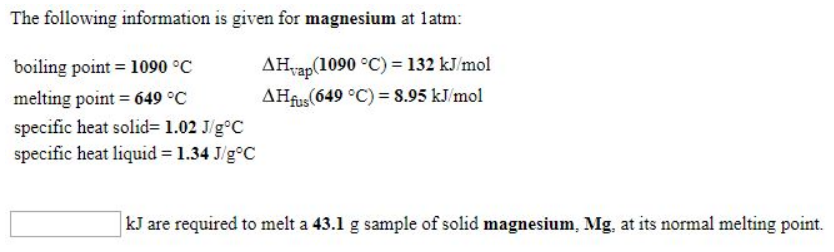# Problem: The following information is given for magnesium at 1 atm: boiling point = 1090°C                        ΔHvap (1090°C) = 132 kJ/molmelting point = 649°C                        ΔHfus (649°C) = 8.95 kJ/molspecific heat solid = 1.02 J/g°C specific heat liquid = 1.34 J/g°C   ________ kJ are required to melt a 43.1 g sample of solid magnesium, Mg, at its normal melting point.

###### FREE Expert Solution
98% (127 ratings)###### Problem Details

The following information is given for magnesium at 1 atm:

boiling point = 1090°C                        ΔHvap (1090°C) = 132 kJ/mol

melting point = 649°C                        ΔHfus (649°C) = 8.95 kJ/mol

specific heat solid = 1.02 J/g°C

specific heat liquid = 1.34 J/g°C

________ kJ are required to melt a 43.1 g sample of solid magnesium, Mg, at its normal melting point.